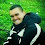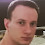# Arbitrary to Decimal Numeral System Conversion in C#

In the article Decimal to Arbitrary Numeral System Conversion in C# I've presented a function that converts a decimal number to a number in an arbitrary numeral system. All you had to do was to call the method passing the decimal number to convert and the radix (a.k.a. base) of the destination numeral system as arguments. Now I'm going to present you a solution to the opposite task - convert a number from an arbitrary to decimal numeral system. The presented implementation can convert any number in an arbitrary numeral system with radix from 2 to 36 to a decimal number.

Using the method is very simple:

``````Console.WriteLine("From Binary : " +
ArbitraryToDecimalSystem("111010110111100110100010101", 2));
Console.WriteLine("From Octal  : " +
ArbitraryToDecimalSystem("726746425", 8));
Console.WriteLine("From Hex    : " +
ArbitraryToDecimalSystem("75BCD15", 16));
Console.WriteLine("From Base 36: " +
ArbitraryToDecimalSystem("21I3V9", 36));

// This example displays the following output:
// From Binary : 123456789
// From Octal  : 123456789
// From Hex    : 123456789
// From Base 36: 123456789``````

Here's the C# source code of the arbitrary to decimal numeral system converter:

``````/// <summary>
/// Converts the given number from the numeral system with the specified
/// radix (in the range [2, 36]) to decimal numeral system.
/// </summary>
/// <param name="number">The arbitrary numeral system number to convert.</param>
/// is in (in the range [2, 36]).</param>
/// <returns></returns>
public static long ArbitraryToDecimalSystem(string number, int radix)
{
const string Digits = "0123456789ABCDEFGHIJKLMNOPQRSTUVWXYZ";

throw new ArgumentException("The radix must be >= 2 and <= " +
Digits.Length.ToString());

if (String.IsNullOrEmpty(number))
return 0;

// Make sure the arbitrary numeral system number is in upper case
number = number.ToUpperInvariant();

long result = 0;
long multiplier = 1;
for (int i = number.Length - 1; i >= 0; i--)
{
char c = number[i];
if (i == 0 && c == '-')
{
// This is the negative sign symbol
result = -result;
break;
}

int digit = Digits.IndexOf(c);
if (digit == -1)
throw new ArgumentException(
"Invalid character in the arbitrary numeral system number",
"number");

result += digit * multiplier;
}

return result;
}``````

1.2.Awesome. Thanks!

3.Hello,

I wanted to let you know that I believe I have found a bug in your code example.

I've been using your ArbitraryToDecimalSystem function for a while (very nice by the way), but I started to notice that my code was not resulting in the expected number of permutations when the arbitrary number contains more than one digit.

For instance if I'm using a base-36 numbering system, "A" returns the expected base-10 value of "10". If I use the number "A1", the function returns the base-10 value of "11" instead of the expected "361". This then causes the inverse conversion to return the incorrect base-36 number (in this example "B").

It appears that your example is missing code related to the multiplier. I added the following to the for loop and it solved the problem.

long multiplier = 1;
if (i < number.Length -1)
{
int exponent = number.Length - 1 - i;
multiplier = Convert.ToInt64(Math.Pow(BaseChars.Length, exponent));
}

Here is a .NET Fiddle I used for my testing: https://dotnetfiddle.net/STDXPu

Cheers!

1.Hi, I tested my original code once again and it is working fine with your example. Please make sure you are calling the method right, for example, if you want to convert the base-36 number "A1" to decimal, you should call the method with the radix parameter set to 36 like this:

long decimalResult = ArbitraryToDecimalSystem("A1", 36);

4.Very valuable snippet, thank you so much for sharing!